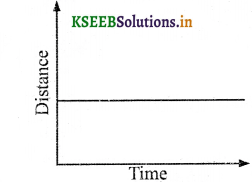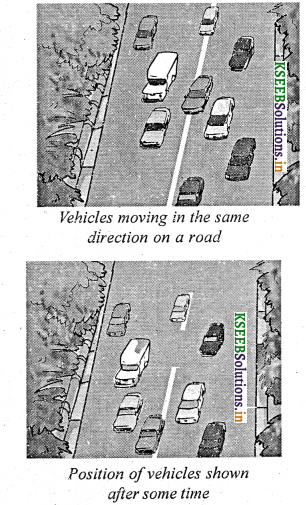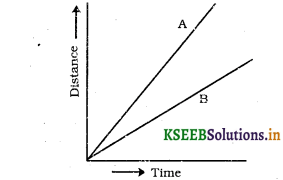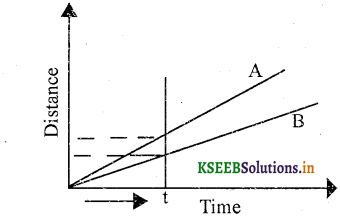# KSEEB Solutions for Class 7 Science Chapter 13 Motion and Time

Students can Download Science Chapter 13 Motion and Time Questions and Answers, Notes Pdf, KSEEB Solutions for Class 7 Science helps you to revise the complete Karnataka State Board Syllabus and to clear all their doubts, score well in final exams.

## Karnataka State Syllabus Class 7 Science Chapter 13 Motion and Time

### Class 7 Science Motion and Time Textbook Exercise Questions and Answers

Question 1.
Classify the following as motion along a straight line, circular or oscillatory motion:

1. The motion of your hands while running,
2. The motion of a horse pulling a cart on a straight road,
3. The motion of a child in a merry – go – round.
4. The motion of a child on a see-saw.
5. The motion of the hammer of an electric bell.
6. The motion of a train on a straight bridge.

1. Oscillatory motion
2. Straight-line motion
3. Circular motion
4. Oscillatory motion
5. Oscillatory motion
6. Straight-line motion.Question 2.
Which of the following are not correct?

1. The basic unit of time is second.
2. Every object moves at a constant speed.
3. Distances between two cities are measured in kilometers.
4. The time period of a given pendulum is constant.
5. The speed of a train is expressed in m/h.

1. Correct
2. Not correct
3. Correct
4. Not correct
5. Not correct

Question 3.
A simple pendulum takes 32S to complete 20 oscillations. What is the time period of the pendulum?
Time is taken = 32 seconds.
Number of oscillations = 20
Time period = ?
$$\text { Time period. }=\frac{\text { Total time taken }}{\text { Number of oscillations }}$$
$$=\frac{32}{20}=\frac{16}{10}=1.6$$ Seconds
∴ The time period of the pendulum is 1.6 seconds.Question 4.
The distance between the two stations is 240 km. A train takes 4 hours to cover this distance. Calculate the speed of the train.
Distance between two stations = 240 km
Time taken = 4 hr
∴ $$\text { Speed }=\frac{\text { Total distance }}{\text { Time taken }}=\frac{240}{4}$$ = 60 km/hr
∴ The speed of the train is 60 km per hour.

Question 5.
The odometer of a car reads 57321,0 km when the clock shows the time 08 : 30 AM. What is the distance moved by car, if at 08:50 AM, the odometer reading has changed to 57336,0 km? Calculate the speed of the car in km/ min during this time. Express the speed in km/h also.
Total distance covered by the car = 57336 – 57321 = 15 km
Initial time = 08.30 AM
Final time =08.50 AM
Time taken = 20 min
$$Speed =\frac{\text { Total distance covered }}{\text { Time taken }}$$
$$=\frac{15}{20}=\frac{3}{4}$$ km per minute = 0.75 x 60 = 45 km/hr
∴ The speed of the car is 45 km per hour.Question 6.
Salma takes 15 minutes from her house to reach her school on a bicycle. If the bicycle has a speed 2m/s, calculate the distance between her house and the school.
Time taken = 15 min = 15 x 60 = 900sec
Speed of the bicycle = 2 m / sec
Distance = Speed x time taken
= 2 x 900
= 1800m
= 1.8 km

∴ The distance between Salma’s house and the school is 1.8 km.

Question 7.
Show the shape of the distance-time graph for the motion in the following cases:
(a) A car moving at a constant speed.
(b) A car parked on a side road.
(a) A car moving with a constant speed covers equal distance in equal intervals of time.(b) A car parked on a side road does not change in distance with an increase in time.Question 8.
Which of the following relations is correct?
(a) Speed = Distance x Time
(b) Speed = $$\frac{\text { Distance }}{\text { Time }}$$
(c) Speed = $$\frac{\text { Distance }}{\text { Time }}$$
(d) Speed = $$\frac{1}{\text { Distance } \times \text { Time }}$$
(b) Speed = $$\frac{\text { Distance }}{\text { Time }}$$

Question 9.
The basic unit of speed is
(a) km/m in
(b) ni/min
(c) km/h
(d) m/s
(d) m/sQuestion 10.
A car moves with a speed of 40 km/ h for 15 minutes and then with a speed of 60 km/h for the next 15 minutes. The total distance covered by the car is:
(a) 100 km
(b) 25 km
(c) 15 km
(d) 10 km
(b) 25 km

Question 11.
Suppose the two photographs, shown below had been taken at an interval of 10 seconds. If a distance of 100 metres is shown by 1 cm in these photographs, calculate the speed of the fastest car.The distance covered by the car from one strip to another, which is measured by scale is 1.4 cm.
Given, 1 cm is equivalent to 100 m
∴ 1.4 cm is equivalent to = 1.4 x 100 = 140 m
∴ Distance travelled by the car = 140 m
Time interval between the two photographs = 10 s
∴ Speed = $$\frac{\text { Distance covered }}{\text { Time taken }}=\frac{140}{10}$$ = 14 m/s
Thus, the speed of the fastest car is 14 m/s.

Question 12.
The figure shows the distance-time graph for the motion of two vehicles A and B. Which one of them is moving faster?Distance – time graph for the motion of two cars.
Speed = $$\frac{\text { Distance covered }}{\text { Time taken }}$$
This relation shows that the speed of a vehicle is greater if it covers maximum distance at a given interval of time. The distance covered is compared by drawing a line perpendicular to the time axis.At a given time t, the distance covered by vehicle A is more than vehicle B.
Hence, vehicle A is moving faster than vehicles.

Question 13.
Which of the following distance-time graphs shows a truck moving with a speed which is not constant?